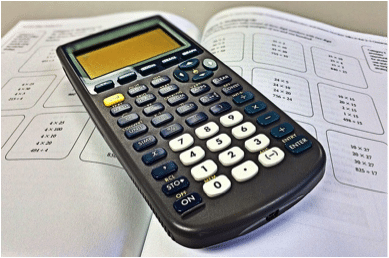# List of Math Tools to Use in the Classroom### The standard at hand is number five: Use appropriate tools strategically.Calculators have been one of the battlefronts in the so-called “Math Wars.” One contingent claims that calculators should be used early and often, and that memorizing number facts is so 1970s; another group claims that any use of calculators hinders the development of conceptual understanding. Some of us seem to be nostalgic for a golden age when kids did all of their math problems using longhand calculations. But the truth is that slide rules were calculators, log tables were calculators, and trig tables were calculators. There have always been shortcuts for tedious calculations (abacus, anyone?). The bar for what kinds of math people should be able to do by hand is in constant motion. No one suggests that kids should know how to calculate the square root of 8,341 by hand. Disagreements on this topic are just a matter of degree, and we have to find the happy medium.

The Common Core curriculum suggests that students should view calculators, computers, tablets, compasses, protractors, rulers, and mental math as elements in their mathematical toolbox to be selected if and when necessary. Students should gain knowledge of various math tools and have an understanding of when they are useful and what their limitations are. Note that this standard says to use tools “strategically.” When kids need to calculate the price of that cool pair of jeans on sale for 20% off, we hope they don’t pull out a phone and type in calculations. Mental math and estimation are often the best and most efficient tools; students should learn when they are appropriate to a situation.

Fluent calculator use implies strong mathematical conceptual knowledge and solid number sense (that can only be gained, really, through time and practice—yup, drill and kill). How many kids have gotten confused when entering the square of a negative number on a calculator? If they enter -42, the calculator returns -16, following the standard order of operations. Some students shrug their shoulders, accepting that the square of -4 is apparently -16. Other students recognize they made a typographical error. If a kid doesn’t really understand the math, it won’t be disguised by handing them a calculator. After all, the calculator only does what we tell it to do.

Conceptual understanding also involves knowing the limits of tools. For example, when graphing functions with vertical asymptotes, some calculators will “connect the dots” and draw a nearly vertical line on the graph. Proficient students will understand that these lines are artifacts of the technology, and not really part of the graph. Proficient students will also understand that if they see an absurdly small number as part of a regression, like 5.4409238E-13, it simply represents the calculator struggling with how to present “0” as a valid regression output.

Calculators are not the only mathematical tools that students should be using. If accessible (not a given for many classrooms), spreadsheets are extremely helpful for statistical calculations and recursive reasoning. Dynamic geometry software can help create visualizations, test theorems, and explore things like polynomial behavior. Compasses, protractors, and physical manipulatives build kinesthetic knowledge of math concepts and help kids relate abstract concepts to the physical world.

Math teachers will continue to need to adapt to new tools and technologies. For example, Wolfram Alpha spits out answers to complex mathematical problems almost instantaneously. Certain smartphone apps can solve problems simply by a user taking a picture of the problem. This technology is only going to accelerate. So, it is more critical than ever that students are skeptical, savvy mathematicians who know the list of math tools at their disposal and can use them confidently to save time, check for errors, and explore concepts.

Oh, and hammers? They will do nicely to help build that Plinko board we’ve been hoping can illustrate Pascal’s triangle. Now, if we can only get our hands on a 3D printer…